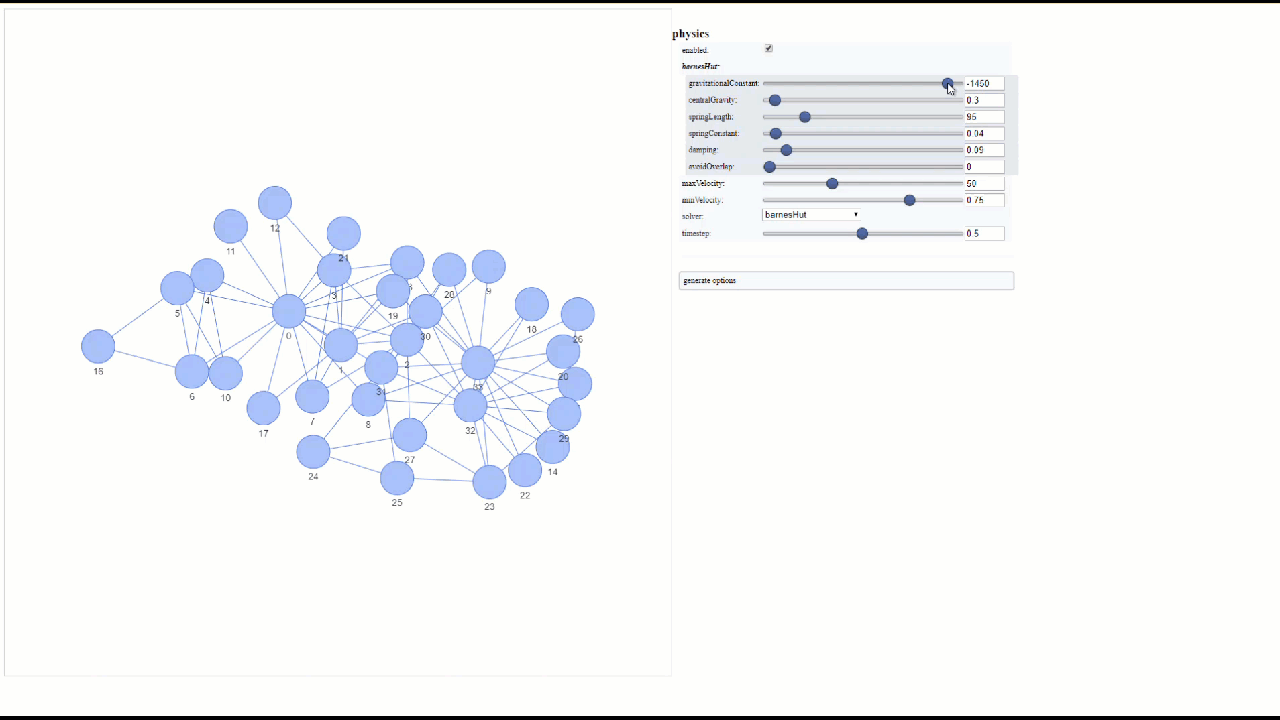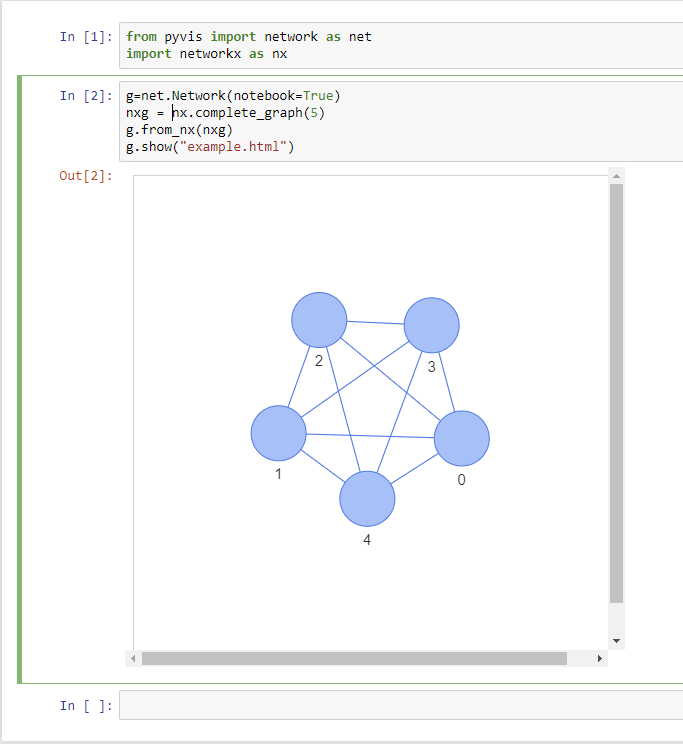# Tutorial¶

The pyvis library is meant for quick generation of visual network graphs with minimal python code. It is designed as a wrapper around the popular Javascript visJS library found at this link.

## Getting started¶

All networks must be instantiated as a `Network` class instance

```>>> from pyvis.network import Network
>>> net = Network()
```

## Add nodes to the network¶

```>>> net.add_node(1, label="Node 1") # node id = 1 and label = Node 1
>>> net.add_node(2) # node id and label = 2
```

Here, the first parameter to the add_node method is the desired ID to give the Node. This can be a string or a numeric. The label argument is the string that will be visibly attached to the node in the final visualization. If no label argument is specified then the node id will be used as a label.

Note

The `ID` parameter must be unique

You can also add a list of nodes

```>>> nodes = ["a", "b", "c", "d"]
>>> net.add_nodes(nodes) # node ids and labels = ["a", "b", "c", "d"]
>>> net.add_nodes("hello") # node ids and labels = ["h", "e", "l", "o"]
```

Note

`network.Network.add_nodes()` accepts any iterable as long as the contents are strings or numerics

## Node properties¶

A call to `add_node()` supports various node properties that can be set individually. All of these properties can be found here, courtesy of VisJS. For the direct Python translation of these attributes, reference the `network.Network.add_node()` docs.

Note

Through no fault of pyvis, some of the attributes in the VisJS documentation do not work as expected, or at all. Pyvis can translate into the JavaScript elements for VisJS but after that it’s up to VisJS!

## Indexing a Node¶

Use the `get_node()` method to index a node by its ID:

```>>> net.add_nodes(["a", "b", "c"])
>>> net.get_node("c")
>>> {'id': 'c', 'label': 'c', 'shape': 'dot'}
```

## Adding list of nodes with properties¶

When using the `network.Network.add_nodes()` method optional keyword arguments can be passed in to add properties to these nodes as well. The valid properties in this case are

```>>> ['size', 'value', 'title', 'x', 'y', 'label', 'color']
```

Each of these keyword args must be the same length as the nodes parameter to the method.

Example:

```>>> g = Network()
title=['I am node 1', 'node 2 here', 'and im node 3'],
x=[21.4, 54.2, 11.2],
y=[100.2, 23.54, 32.1],
label=['NODE 1', 'NODE 2', 'NODE 3'],
color=['#00ff1e', '#162347', '#dd4b39'])
```

Note

If you mouse over each node you will see that the `title` of a node attribute is responsible for rendering data on mouse hover. You can add `HTML` in your `title` string and it will be rendered as such.

Note

The `color` attribute can also be a plain HTML `color` like red or blue. You can also specify the full `rgba` specification if needed. The VisJS documentation has more details.

Detailed optional argument documentation for nodes are in the `network.Network.add_node()` method documentation.

## Edges¶

Assuming the network’s nodes exist, the edges can then be added according to node id’s

```>>> net.add_node(0, label='a')
```

Edges can contain a `weight` attribute as well

```>>> net.add_edge(0, 1, weight=.87)
```

Edges can be customized and documentation on options can be found at `network.Network.add_edge()` method documentation, or by referencing the original VisJS edge module docs.

## Networkx integration¶

An easy way to visualize and construct pyvis networks is to use Networkx and use pyvis’s built-in networkx helper method to translate the graph. Note that the Networkx node properties with the same names as those consumed by pyvis (e.g., `title`) are translated directly to the correspondingly-named pyvis node attributes.

```>>> from pyvis.network import Network
>>> import networkx as nx
>>> nx_graph = nx.cycle_graph(10)
>>> nx_graph.nodes['title'] = 'Number 1'
>>> nx_graph.nodes['group'] = 1
>>> nx_graph.nodes['title'] = 'I belong to a different group!'
>>> nx_graph.nodes['group'] = 10
>>> nx_graph.add_node(25, size=25, label='lonely', title='lonely node', group=3)
>>> nt = Network('500px', '500px')
# populates the nodes and edges data structures
>>> nt.from_nx(nx_graph)
>>> nt.show('nx.html')
```

## Visualization¶

The displaying of a graph is achieved by a single method call on `network.Network.show()` after the underlying network is constructed. The interactive visualization is presented as a static HTML file.

```>>> net.enable_physics(True)
>>> net.show('mygraph.html')
```

Note

Triggering the `toggle_physics()` method allows for more fluid graph interactions

## Example: Visualizing a Game of Thrones character network¶

The following code block is a minimal example of the capabilities of pyvis.

```from pyvis.network import Network
import pandas as pd

got_net = Network(height='750px', width='100%', bgcolor='#222222', font_color='white')

# set the physics layout of the network
got_net.barnes_hut()

sources = got_data['Source']
targets = got_data['Target']
weights = got_data['Weight']

edge_data = zip(sources, targets, weights)

for e in edge_data:
src = e
dst = e
w = e

# add neighbor data to node hover data
for node in got_net.nodes:
node['title'] += ' Neighbors:<br>' + '<br>'.join(neighbor_map[node['id']])
node['value'] = len(neighbor_map[node['id']])

got_net.show('gameofthrones.html')
```

If you want to try out the above code, the csv data source can be downloaded

Note

The `title` attribute of each node is responsible for rendering data on node hover.

## Using the configuration UI to dynamically tweak `Network` settings¶

You also have the option of supplying your visualization with a UI used to dynamically alter some of the settings pertaining to your network. This could be useful for finding the most optimal parameters to your graph’s physics and layout function.

```>>> net.show_buttons(filter_=['physics'])
```Note

You can copy/paste the output from the generate options button in the above UI into `network.Network.set_options()` to finalize your results from experimentation with the settings.## Using pyvis within Jupyter notebook¶

Pyvis supports Jupyter notebook embedding through the use of the `network.Network()` constructor. The network instance must be “prepped” during instantiation by supplying the notebook=True kwarg. Example: